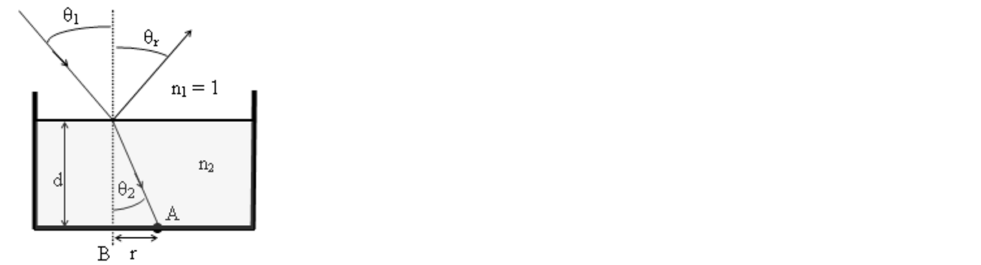Problem: As shown at right below, a laser is directed into a water tank (filled with water having an index of refraction n2 at the laser wavelength) at an angle  θ1 from the vertical and strikes the bottom of the tank at point A, a horizontal ditance r away from B, the spot directly underneath the place where the light enters the water. The index of refraction of water for red light is 1.331, while the index of refraction of water for green light is 1.336. The angle of refraction θ2 would bea) the same for a green and a red laser.b) smaller for a red laser than for a green laser.c) smaller for a green laser than for a red laser.

FREE Expert Solution
88% (404 ratings)
Problem Details

As shown at right below, a laser is directed into a water tank (filled with water having an index of refraction nat the laser wavelength) at an angle  θ1 from the vertical and strikes the bottom of the tank at point A, a horizontal ditance r away from B, the spot directly underneath the place where the light enters the water. The index of refraction of water for red light is 1.331, while the index of refraction of water for green light is 1.336. The angle of refraction θ2 would be

a) the same for a green and a red laser.

b) smaller for a red laser than for a green laser.

c) smaller for a green laser than for a red laser.